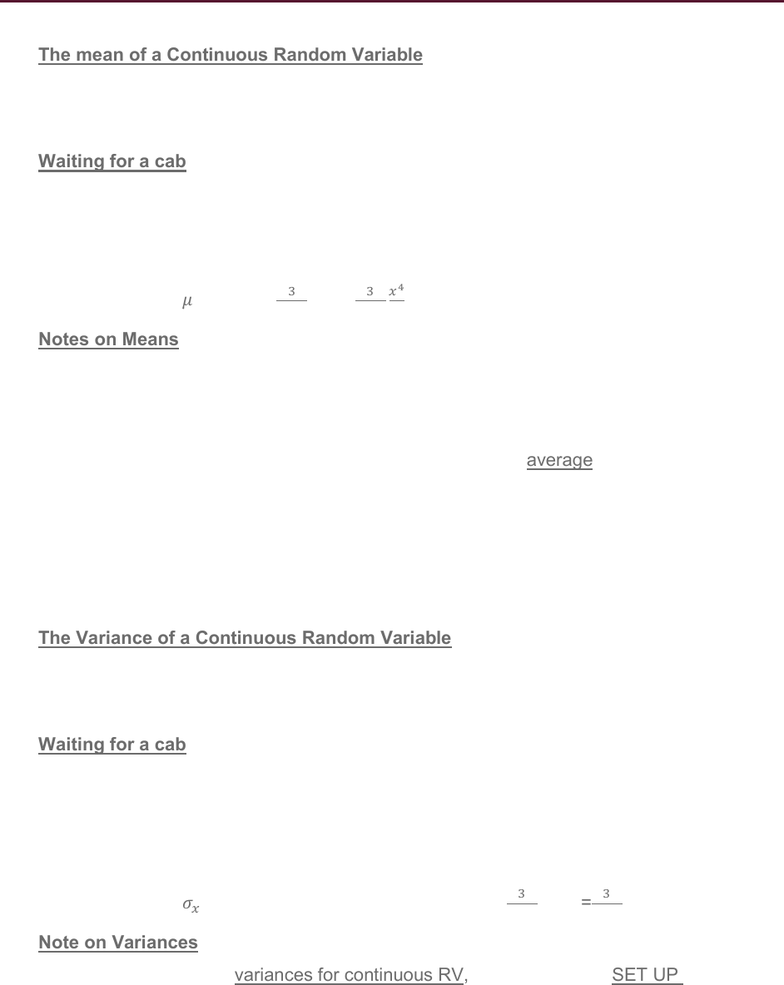# STAT 1430 Lecture Notes - Lecture 17: Probability Distribution, Random Variable, Standard Deviation

82 views3 pagesSTAT1430.01Lecture17Random Variable March 6, 2019
The mean of a Continuous Random Variable
o Notation:
o Formula: 

Waiting for a cab
o Suppose X=time waiting for a cab, where you will only wait up to 10 minutes.
o Suppose f(x)=3/1000 * 2 where [0<X<10]
o Find the mean waiting time. Include units.



 
Notes on Means
o Always integrate over entire interval for X
o Don’t forget to put the “x” in your integral. What would the result be if you forgot
the x?
o Your answer is not a probability. It’s the expected average value of X.
possible values of x.
o The mean of X is in units of X.
o We expect you to be able to set up and calculate the mean for both discrete and
continuous random variables.
The Variance of a Continuous Random Variable
o Notation:
o Formula: 


Waiting for a cab
o Suppose X = time waiting for a cab, where you will only wait up to 10 minutes.
o Suppose f(x) = 3/1000 x2 where [0 < X < 10]
o SET UP the variance of the waiting times. Include all details, such as the value of
the mean.
=
 

=


Note on Variances
o When finding variances for continuous RV, you only have to SET UP the
integrals, not calculate them out. (See examples for details)
Unlock document

This preview shows page 1 of the document.
Unlock all 3 pages and 3 million more documents.

## Get access

\$8 USD/m\$10 USD/m
Billed \$96 USD annuallyHomework Help
Study Guides
Textbook Solutions
Class Notes
Textbook Notes
Booster Class